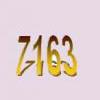#### You may also like### Multiplication Magic

Given any 3 digit number you can use the given digits and name another number which is divisible by 37 (e.g. given 628 you say 628371 is divisible by 37 because you know that 6+3 = 2+7 = 8+1 = 9). The question asks you to explain the trick.### Old Nuts

In turn 4 people throw away three nuts from a pile and hide a quarter of the remainder finally leaving a multiple of 4 nuts. How many nuts were at the start?### Some Cubes

The sum of the cubes of two numbers is 7163. What are these numbers?

# Sixational

##### Age 14 to 18 Challenge Level:

The $n$th term of a sequence is given by the formula

$n^3 + 11n$.

Find the first four terms of the sequence given by this formula and the first term of the sequence which is bigger than one million.

Prove that all terms of the sequence are divisible by $6$.